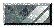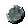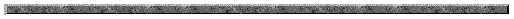Triangle Area CalculatorBack to Activities page

The typical formula for calculating the area of a triangle is 1/2(Base)*(Height) which many people describe as one-half the base of the triangle times the height.

Example: A triangle with base 3 feet and height 4 feet has an area of 1/2*3ft*4ft = 1/2 * 12(ft^2) = 6 (ft^2) = 6 square feet.

Sometimes we are not given the base and the height and it might be more difficult to calculate the area. However, what if we were given the measurements of the three sides of the triangle?  Could we calculate the area? It turns out that we can.

A formula for calcualting the area of a triangle when all sides are known is attirbuted to two famous mathematicians; Heron of Alexandria and Archimedes.

A triangle has sides a, b, and c.
After Calculating S, where S = (a+b+c)/2
The Area of a Triangle = SQRT(s*(s-a)(s-b)(s-c))
Use the Tool Below to calculate the Area of a Triangle with three
side lengths A,B, and C.

REMEMBER: Square your Units !!!

Heron's Formula for Calculating the Area of a Triangle

 Side Lengths A = B = C =
 or Area of Triangle = S = (A+B+C)/2 =>

OOooops: Did you get a message about the Triangle Inequality Theorem? What is that?

The triangle inequality theorem basically says that all three sides of a triangle have to meet. Imagine a triangle with side length 3 feet, 4 feet, and 150 feet. The two shorter sides cannot reach the ends of the longest side. Therefore it is not a triangle.

The triangle inequality theorem states more clearly:

The SUM of TWO SIDES MUST ADD UP TO BE GREATER THAN THE LENGTH OF THE REMAINING THIRD SIDE.

OR (with sides A,B,C):

• A + B > C,
• B + C> A,
• and A + C>B

That having been established can you have a triangle with sides 5, 12, 16?

How about 5, 12, 13? What does this look like?

Check out the Pythagorean Thoerem for more information?Welcome Page| Activities| Program Area Links| Standards Alignment | Description of Tutorials

Email Jim Dildine